# Motion Planning Solution with Constraints Based on Minimum Distance Model for Lane Change Problem of Autonomous Vehicles

Motion Planning Solution with Constraints Based on Minimum Distance Model for Lane Change Problem of Autonomous Vehicles
University of Arts, Hue University, Hue 530000, Vietnam

Vietnam - Korea University of Information and Communication Technologies, Da Nang University, Da Nang 550000, Vietnam

Faculty of Information Technology, University of Education, Da Nang University, Da Nang 550000, Vietnam

Corresponding Author Email:
qhaitho@hueuni.edu.vn
Page:
251-260
|
DOI:
https://doi.org/10.18280/mmep.090131
9 September 2021
|
Accepted:
13 January 2022
|
Published:
28 February 2022
| Citation

OPEN ACCESS

Abstract:

Lane change is one of the important operations in motion of an autonomous vehicle. When encountering obstacles or wanting to overtake the vehicle ahead, the autonomous vehicle will make a decision and choose the best path to control the trajectory of motion to perform lane change. In this article, we will present solutions for lane change trajectories, including general path setting, building nonlinear models with states of vehicle speed, acceleration and jerk; building a constraint set to avoid collisions with a minimum safe distance model, which takes into account the potentially collision angle positions during lane change. Simulation results are performed in Matlab simulation environment to demonstrate an effective proposed solution and addressed the disadvantages in the modeling process for lane-changing operations, in order to improve the proactive safety of the motion planning for autonomous vehicles.

Keywords:

autonomous vehicle, path planning, lane change, motion planning, intelligent transportation systems

1. Introduction

The signals obtained from the equipment of the radar sensor system, laser waves, cameras, LiDAR are transferred to the processing device to decrypt the information obtained, and from which it will be converted into a command for the autonomous vehicle to perform actions such as turning and entering lanes, overcoming obstacles, which makes it convenient to move.

Lane change is a complex process for motion planning for autonomous vehicles. Improper lane change can cause accidents and traffic congestion. According to incomplete statistics, the number of accidents in which the driver manipulates the lane change incorrectly accounts for 5-10% of the total number of traffic accidents , the safety of the transport system is affected by the types of vehicles, location, speed, the ability to increase/decrease the speed of vehicles when participating in traffic encountering obstacles in the moving lane is a problem that needs to be studied.

In the problem of motion planning for Autonomous vehicles (AV), the trajectory is built with many different methods, different types of geometric roads with different operational characteristics in terms of continuity, smooth and slippery. Geometric types of roads are commonly applied when building trajectory such as the Bezier line [2-5], the spline [6-10], the acceleration curve [11-15], and the polynomial curve [16-21]. Therefore, choosing a suitable geometric line to represent the motion trajectory of the autonomous vehicle in complex environmental conditions is very important.

The disadvantages in this problem are that the models of motion trajectory focus only on lane change or automatically overtake, ignoring the popularity of the model for lane change and overtaking operations. As well as the operating states of the Autonomous vehicle are not carefully considered, from which it in turn leads to uncontrolled cases so collisions can occur. Finally, suppose the surroundings, the speed of vehicles or the number of vehicles around them are not suitable, namely assuming that the surroundings are static and autonomous vehicles operate, or assuming the speed of vehicles involved in traffic is constant. In order to overcome the disadvantages, in this study, a solution is built to establish a motion trajectory based on optimizing the nonlinear model, of which the trajectory is established based on the structure of appropriate geometric roads and the current traffic state. With constraints being the angular points, capable of collision during lane change, determining these constraints is carried out to determine the actual distance model of autonomous vehicles and other vehicles involved in traffic, then with a minimum safe distance to make a decision whether to perform lane change and avoid obstacles. The purpose of this solution is to improve the active safety of the motion planning process for autonomous vehicles when participating in traffic.

The next part of the paper will introduce the vehicle's approach to establishing lane motion trajectories, including setting a general path, building a nonlinear model with the states of velocity, acceleration, and jerk (the variable degree of acceleration overtime) of the vehicle, building a set of constraints to avoid collisions with the minimum safe distance model, which will take into account the angular positions that are likely to collide during lane change. Followed is an experimental and concluding section with some proposals for further research for the autonomous vehicle problem.

2. Building an Trajectory for Lane Change Solution

Lane change is one of the important operations in the movement of the autonomous vehicle. When encountering obstacles or wanting to overtake the vehicle in front, the autonomous vehicle will make a decision and choose the best path to control the trajectory of the movement to perform lane change.

The proposed lane change solution was carried out in four Phases (Figure 1), including:

- Phase 1: Locate the position of the autonomous vehicle and surrounding objects through GPS positioning data, sensor system installed on the vehicle.

- Phase 2: Build the trajectory of the initial lane change operation.

- Phase 3: Determine the Target function and the constraints to make a decision on how to change lanes ensuring safety, avoiding collisions and that the motion trajectory is smooth and slippery.

- Phase 4: Determine the parameters to create the final motion trajectory.

## 1.jpg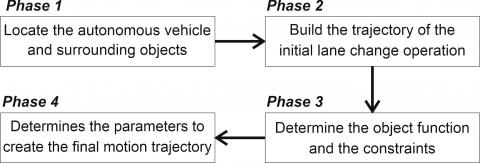Figure 1. Phases of lane change solutions for autonomous vehicle

This article only solves the problem of lane change with the case of an autonomous vehicle moving along a straight road having a lane line for overtaking, the process of determining the trajectory is carried out with the model of road, vehicle (Figure 2) and other objects in the overall Cartesian coordinate system (x,y), in which x-axis is the vertical direction and the y axis is the horizontal direction of the road. Each vehicle and other objects are described by their positions (Sx,Sy) in the lane's boundary. Representatives for the system state include the directional angle θr, distance from the boundary to the vehicle dr, the reference arc length Sr and Kr (Sr) are the circular arc curvature parameter of the reference curve . Therefore, it is necessary to process the geodesy coordinate data obtained from GPS devices to Cartesian coordinate data.

## 2.jpg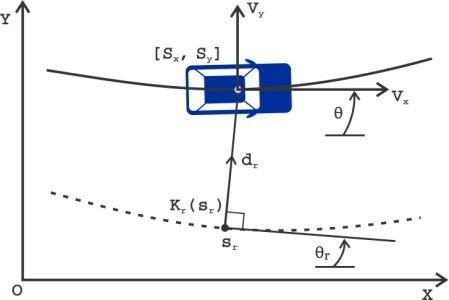Figure 2. Vehicle model and reference curve

2.1 Locating position of the objects from GPS data

The GPS system provides three-dimensional coordinates of the geodetic coordinate system including: latitude B, longitude L and altitude H. These coordinates are calculated according to the WGS-84 coordinate system, which makes it difficult for the data obtained from the GPS to directly reflect the vehicle's movement in the Cartesian coordinate system, the one that this article uses to solve the given problem. Therefore, in order for the data obtained from the GPS device to accurately describe the movement of a human-controlled vehicle, it needs to convert the coordinates of the geodetic coordinate system (B, L, H) into Cartesian coordinate system (X, Y, Z) (Figure 3).

## 3.png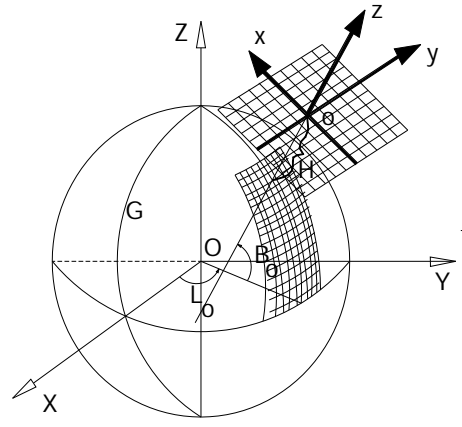Figure 3. Relationship between coordinate systems

The data obtained from the GPS device includes values (B, L, H), to convert between geocentric coordinates XYZ and geocentric coordinates xyz, we use the following formula :

$\left[\begin{array}{l} X \\ Y \\ Z \end{array}\right]=\left[\begin{array}{ccc} -\sin B \cos L & -\sin B \sin L & \cos B \\ -\sin L & \cos L & 0 \\ \cos B \cos L & \cos B \sin L & \sin B \end{array}\right]\left[\begin{array}{l} x \\ y \\ z \end{array}\right]+\left[\begin{array}{c} (N+H) \cos B \cos L \\ (N+H) \cos B \sin L \\ {\left[N\left(1-e^{2}\right)+H\right] \sin B} \end{array}\right]$            (1)

$\left[\begin{array}{l} x \\ y \\ z \end{array}\right]=\left[\begin{array}{ccc} -\sin B \cos L & -\sin B \sin L & \cos B \\ -\sin L & \cos L & 0 \\ \cos B \cos L & \cos B \sin L & \sin B \end{array}\right] \cdot\left[\begin{array}{c} X-(N+H) \cos B \cos L \\ Y-(N+H) \cos B \sin L \\ Z-\left[N\left(1-e^{2}\right)+H\right] \sin B \end{array}\right]$            (2)

where: B, L, H are geodetic coordinates of the grid center point or the origin of the selected geographical coordinate system such that x, y, z are always positive; N is the first vertical radius of curvature passing through the origin of the geocentric coordinate system.

$N=\frac{a^{2}}{\sqrt{a^{2} \cos ^{2} B+b^{2} \sin ^{2} B}}$            (3)

where: a is the radius of the large axis and b is the radius of the small axis of the Ellipsoid WGS-84 (a = 6378137.0000 and b = 6356863.0188); e is the first false mind of Ellipsoid; X, Y, Z are the coordinates perpendicular to the geocentric space of the point to be shifted; x, y, z are the coordinates perpendicular to the geospatial space of the point to be converted.

Due to the problem locating the motion trajectory only focuses on the plane coordinate components (X, Y) and (B, L) (but not on the altitudes Z and H), the formulas converting coordinates will not mention the altitude Z and H. Therefore, at a point of geodetic coordinates (B, L) when converting into the coordinates of the right angle (X, Y), it will be calculated by the formula :

$\left\{\begin{array}{l} X=(N+H) \cos B \cos L \\ Y=(N+H) \cos B \sin L \end{array}\right.$            (4)

2.2 Building a lane change trajectory equation

The trajectory motion is a common problem in the operation control of the autonomous vehicle. To complete the given motion planning problem, the vehicle must move in the correct trajectory. In other words, trajectory is the basic element to describe the operation of the autonomous vehicle. The design of the moving trajectory will provide input data for the control system, and also be the direct basis for the operation control of the autonomous vehicle.

The problem of building the motion trajectory will involve issues of dynamics and dynamics, which include the geometric elements of the moving path, along with the time elements performing motion such as acceleration and velocity. Therefore, in this study, we select the Quintic polynomial equation to build the original lane change trajectory. We use this polynomial function because the third derivative equation will create a continuous, smooth and slippery trajectory.

Polynomial curve is used to build lane change trajectory with the polynomial equations is set as follows:

$y(x)=b_{5} x^{5}+b_{4} x^{4}+b_{3} x^{3}+b_{2} x^{2}+b_{1} x+b_{0}$           (5)

where: x represents the vertical position and y represents the horizontal position of the vehicle in the XY coordinate axle system. b5,...,b0 are unknown values and need to be calculated.

In the road structure (Figure 4), the road system is determined by adjacent lanes of arbitrary shape and curvature. In this article, for the convenience of performance, we assume and consider the (lanei ) to be a path defined by the left (BLi ) and right border (BRi ). Such a path is defined as a polyline and a combination of all lanes at a certain time period (lane(s)=∪i lanei), and know in advance the relationship between adjacent lanes.

## 4.jpg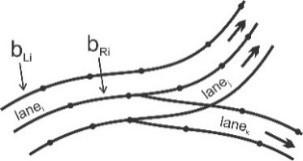For lane change, with the information received from the sensor, from GPS ..., the equation representing the horizontal position yst, vertical position xst, cst derivative point is the first derivative of yst and the curvature kst is the second derivative of the current lane yst in the Cartesian coordinate system, the starting position for the construction of the lane change trajectory established as follows:

$y_{s t}=b_{5} x_{s t}{ }^{5}+b_{4} x_{s t}{ }^{4}+b_{3} x_{s t}{ }^{3}+b_{2} x_{s t}{ }^{2}+b_{1} x_{s t}+b_{0}$          (6)

$c_{s t}=5 b_{5} x_{s t}^{4}+4 b_{4} x_{s t}^{3}+3 b_{3} x_{s t}^{2}+2 b_{2} x_{s t}+b_{1}$          (7)

$k_{s t}=20 b_{5} x_{s t}{ }^{3}+12 b_{4} x_{s t}{ }^{2}+6 b_{3} x_{s t}+2 b_{2}$          (8)

And the equation representing horizontal position yed, vertical position xed, derivative point ced is the first derivative of yed and curvature ked is the second derivative yed of the next lane at the end position of the lane change trajectory set as follows:

$y_{e d}=b_{5} x_{e d}{ }^{5}+b_{4} x_{e d}{ }^{4}+b_{3} x_{e d}{ }^{3}+b_{2} x_{e d}{ }^{2}+b_{1} x_{e d}+b_{0}$          (9)

$c_{e d}=5 b_{5} x_{e d}^{4}+4 b_{4} x_{e d}^{3}+3 b_{3} x_{e d}^{2}+2 b_{2} x_{e d}+b_{1}$          (10)

$k_{e d}=20 b_{5} x_{e d}^{3}+12 b_{4} x_{e d}^{2}+6 b_{3} x_{e d}+2 b_{2}$          (11)

From the Eqns. (6)-(11), with the establishment of b5, b4, b3, b2, b1, b0 values will create the corresponding lane change trajectory for the motion planning process of the autonomous vehicle.

For obstacle trajectory, the implementation solution is similar to creating a lane change trajectory, but the different point of the obstacle trajectory will be a combination of 2 lane change trajectory (Figure 5).

## 5.jpg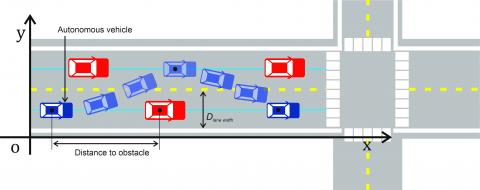Figure 5. Lane change trajectory

Thus, with the information obtained from the surrounding environment, the decision on lane change or obstacle overtaking behavior will be made with the trajectory created by the Quintic polynomial Equation curve as presented; the unknown parameters b5, b4, b3, b2, b1, bof the trajectory equation will be the first values of the system. These values will change to suit the different operating environment, the use of polynomial Equation to create a path trajectory to exploit the advantage of continuous derivatives and curvature of the line created by this method achieves smooth and slippery. In addition, creating this lane change trajectory can also apply different types of roads and methods depending on the traffic situation.

2.3 Identifying object functions

In the process of building a solution to establish a motion trajectory, factors need to be calculated and considered such as dynamics systems, constraint set in the operation of the vehicle, environmental limitations such as the structure of the road system, obstacles. The goal of the problem is to find a safe and viable trajectory. In this article, we propose an approach solution that uses Model Predictive Control (MPC) to carry out motion trajectory planning, in which the trajectory is expected to be updated in the next stages of the model and the construction of the cost function with collision avoidance constraints for the problem of creating optimal trajectory motion.

The first part of the nonlinear vehicle model with state vectors including: s(x) reference location, j(x) jerk, a(x) acceleration and v(x) vehicle velocity is recommended as follows:

$s(x)=b_{5} x^{5}+b_{4} x^{4}+b_{3} x^{3}+b_{2} x^{2}+b_{1} x+b_{0}$          (12)

$v(x)=\dot{S}(x)$          (13)

$a(x)=\ddot{s}(x)$          (14)

$j(x)=\dddot s(x)$          (15)

Corresponding to:

$v(x)=5 b_{5} x^{4}+4 b_{4} x^{3}+3 b_{3} x^{2}+2 b_{2} x+b_{1}$          (16)

$a(x)=20 b_{5} x^{3}+12 b_{4} x^{2}+6 b_{3} x+2 b_{2}$          (17)

$j(x)=60 b_{5} x^{2}+20 b_{4} x+6 b_{3}$          (18)

where, the parameters b5,...,b0 are unknown and need to be determined.

At the starting position of the lane change trajectory, the initial values of s(0) the reference position, a(0) acceleration, and v(0) velocity at the base of the coordinate system are calculated as follows:

$s(0)=0$          (19)

$v(0)=0$          (20)

$a(0)=0$          (21)

The solution in this paper is to build a time-bound optimization that is commonly discrete at m time step and tk used to denote the end of each time period, with a time limit $\tau=\sum_{k=1}^{m} \Delta t_{k}$.

At the initial time t0=0, s0=0 the position of the vehicle on the coordinate axis system is determined at the point (x0,y0), then at the time $t_{i}$ the vehicle position at (xi,yi) coordinates and the si reference value on the coordinate axis system is described by a discrete time model with calculus integral as follows:

$s_{i}=\int_{0}^{x_{i}} \sqrt{1+\left(\frac{d y}{d x}\right)^{2}} d t$          (22)

In order to achieve the optimal motion trajectory, it is necessary to process the cost function to the minimum value and at the same time the constraints need to be separated from each other. For lane change and obstacle overtaking operations in this study, the idea of handling was to ensure the vehicle's speed of motion and jerk at optimal value. Moreover, the collision avoidance warning situation and also the constraint to decide whether to perform a lane change or obstacle overtaking should be activated before the autonomous vehicle is too close to the obstacle, if in the case of the vehicle has come too close, the ability to miscalculate the cognitive coefficient and forecasting will be uncertain, and if the deviation is small both in position and direction from the reference curve will minimize the possibility of collision with the vehicles ahead, helping the autonomous system to control the trajectory motion.

As such, the J cost function will be built by combining components including acceleration, jerk for a limited time period $\tau \text { and } \tau$ divided into the following I time periods:

$\min J=\frac{1}{m} \sum_{i=1}^{m} a_{i}^{2}+\frac{1}{m} \sum_{i=1}^{m} j_{i}^{2}+\tau^{2}$          (23)

where: ai is the acceleration and ji is the jerk of the vehicle at the time ti, τ is the limited amount of time of the entire process of performing lane change.

To avoid possible collisions, autonomous vehicles must maintain a safe distance from surrounding vehicles during lane changes or overtaking. However, in order to improve the effectiveness of the solution, the constraint set before making a lane change decision needs to be considered as potentially collision angle locations, thereby determining the real distance model of the autonomous vehicle and surrounding vehicles. The minimum safety distance and the risks that will occur during the lane change will be analyzed, and the motion planning process will adjust the state to improve the vehicle's active safety.

2.4 Building a minimum safe distance model to avoid collisions

When changing lanes or overtaking the vehicle in front, there are 04 possible collisions (Figure 6): a) collision with the vehicle in the lower left corner, b) collision with the vehicle in the front left corner, c) collision with the vehicle in the right rear corner and d) collision with the vehicle in the front right corner. The cause of the collision is: i) the speed of the vehicle in front decreases suddenly and ii) the speed of the vehicle behind is greater than the speed of the vehicle in front.

This paper, based on the principle of dynamic modeling of autonomous vehicles, will build a model of the minimum safe distance between vehicles to handle 04 situations stated above. The minimum safety is known to be the necessary distance between vehicles to avoid collisions.

To solve this problem, we need to make the following assumptions:

- The vehicles in front and behind of autonomous vehicles (VIH_LF, VIH_RF, VIH_LB, VIH_RB) move vertical the road, in the same direction as the autonomous vehicle (VIH_AV) and the speed of these vehicles is constant.

The coordinates of the objects are determined by the surrounding rectangle and the position of the object is the center of the corresponding rectangle (Figure 7).

The process of performing lane change or overtaking is calculated in the Cartesian coordinates system, O(0,0) the root coordinates at the center of the vehicle the last obstacle (Figure 6).

## 6.jpg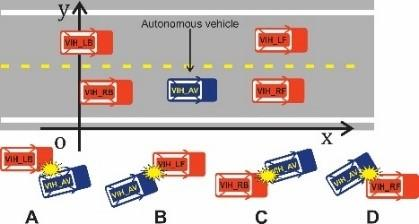Figure 6. Vehicle model in Cartesian coordinate system and collision states

## 7.jpg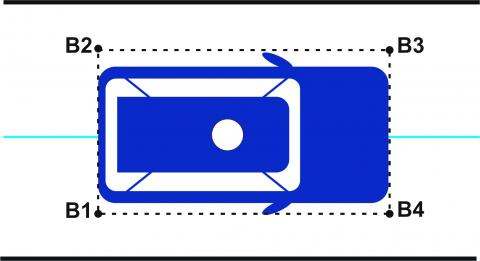Figure 7. The location of the vehicle's defining coordinates

The speed of each vehicle is likely to change during the movement, so the start time of lane change will be determined as the first time with a value of zero. Therefore, the location of each vehicle and the parameters used in the calculation are as follows:

Autonomous vehicle (VIH_AV): The vehicle's current location (xav,yav), the vehicle initial position $\left(x_{0_{-} a v}, y_{0_{-} a v}\right)$, vehicle length Lav, vehicle width Wav, current and initial speed of vehicle vav, v0_av and the acceleration of the vehicle aav

With the current position (xav,yav):

\begin{aligned} &x_{a v}=x_{0_{-} a v}+\int\left(v_{0_{-} a v, x}+\int a_{a v, x} d t\right) d t \\ &y_{a v}=y_{0_{-} a v}+\int\left(v_{0_{-} a v, y}+\int a_{\alpha v, y} d t\right) d t \end{aligned}          (24)

Coordinates of the 4 rectangular corners surrounding the vehicle VIH_AV:

\begin{aligned} &B_{1, \alpha v}\left(x_{a v}-\frac{L_{\alpha v}}{2}, y_{\alpha v}-\frac{\mathrm{W}_{\alpha v}}{2}\right), B_{2, a v}\left(x_{\alpha v}-\frac{L_{\alpha v}}{2}, y_{\alpha v}+\frac{\mathrm{W}_{\alpha v}}{2}\right), \\ &B_{3, a v}\left(x_{\alpha v}+\frac{L_{\alpha v}}{2}, y_{\alpha v}-\frac{\mathrm{W}_{\alpha v}}{2}\right), B_{4, a v}\left(x_{\alpha v}+\frac{L_{\alpha v}}{2}, y_{\alpha v}+\frac{\mathrm{W}_{\alpha v}}{2}\right). \end{aligned}

The front vehicle on the right (VIH_RF): The current position of the vehicle (xrf,yrf ), the initial position of the vehicle $\left(x_{0_{-} r f}, y_{0_{-} r f}\right)$, the length of the vehicle Lrf, the width of the vehicle Wrf, the current and init velocity of the vehicle vrf, v0_rf; and the acceleration of the vehicle arf

With the current position (xrf,yrf ):

\begin{aligned} &x_{r f}=x_{0_{-} r f}+\int\left(v_{0_{-} r f, x}+\int a_{r f, x} d t\right) d t \\ &y_{r f}=y_{0_{-} r f}+\int\left(v_{0_{-}} r f, y+\int a_{r f, y} d t\right) d t \end{aligned}           (25)

Coordinates of the 4 rectangular corners surrounding the vehicle VIH_RF:

\begin{aligned} &B_{1, r f}\left(x_{r f}-\frac{L_{r f}}{2}, y_{r f}-\frac{\mathrm{W}_{r f}}{2}\right), B_{2, r f}\left(x_{r f}-\frac{L_{r f}}{2}, y_{r f}+\frac{\mathrm{W}_{v f}}{2}\right), \\ &B_{3, r f}\left(x_{r f}+\frac{L_{r f}}{2}, y_{r f}-\frac{\mathrm{W}_{r f}}{2}\right), B_{4, r f}\left(x_{r f}+\frac{L_{r f}}{2}, y_{r f}+\frac{\mathrm{W}_{v f}}{2}\right) . \end{aligned}

The front vehicle on the left (VIH_LF): The current position of the vehicle (xlf,ylf ), The initial position of the vehicle $\left(x_{0_{-} l f}, y_{0_{-} l f}\right)$, the length of the vehicle Llf, the width of the vehicle Wlf, the current and initial velocity of the vehicle vlf, v0_lf; and the acceleration of the vehicle alf

With the current position (xlf,ylf ):

\begin{aligned} &x_{l f}=x_{0_{-} l f}+\int\left(v_{0_{-} l f, x}+\int a_{l f, x} d t\right) d t \\ &y_{l f}=y_{0_{-} l f}+\int\left(v_{0_{-} l f, y}+\int a_{l f, y} d t\right) d t \end{aligned}           (26)

Coordinates of the 4 rectangular corners surrounding the vehicle VIH_LF:

\begin{aligned} &B_{1, l f}\left(x_{l f}-\frac{L_{l f}}{2}, y_{l f}-\frac{\mathrm{W}_{l f}}{2}\right), B_{2, l f}\left(x_{l f}-\frac{L_{l f}}{2}, y_{l f}+\frac{\mathrm{W}_{l f}}{2}\right), \\ &B_{3, l f}\left(x_{l f}+\frac{L_{l f}}{2}, y_{l f}-\frac{\mathrm{W}_{l f}}{2}\right), B_{4, l f}\left(x_{l f}+\frac{L_{l f}}{2}, y_{l f}+\frac{\mathrm{W}_{l f}}{2}\right) . \end{aligned}

The behind vehicle on the right (VIH_RB): the current position of the vehicle (xrb,yrb), the initial position of the vehicle $\left(x_{0_{-} r b}, y_{0_{-} r b}\right)$, the length of the vehicle Lrb, the width of the vehicle Wrb, the current and original velocity of the vehicle vrb, v0_rb; and the acceleration of the vehicle arb

With the current position (xrb,yrb ):

\begin{aligned} &x_{r b}=x_{0_{-} r b}+\int\left(v_{0_{-} r b_{, x}}+\int a_{r b_{, x}} d t\right) d t \\ &y_{r b}=y_{0_{-} r b}+\int\left(v_{0_{-} r b_{, y}}+\int a_{r b_{, y} y} d t\right) d t \end{aligned}          (27)

Coordinates of the 4 rectangular corners surrounding the vehicle VIH_RB:

\begin{aligned} &B_{1, r b}\left(x_{r b}-\frac{L_{r b}}{2}, y_{r b}-\frac{\mathrm{W}_{r b}}{2}\right), B_{2, r b}\left(x_{r b}-\frac{L_{r b}}{2}, y_{r b}+\frac{\mathrm{W}_{r b}}{2}\right), \\ &B_{3, r b}\left(x_{r b}+\frac{L_{r b}}{2}, y_{r b}-\frac{\mathrm{W}_{r b}}{2}\right), B_{4, r b}\left(x_{r b}+\frac{L_{r b}}{2}, y_{r b}+\frac{\mathrm{W}_{r b}}{2}\right) . \end{aligned}

The rear vehicle on the left (VIH_LB): (xlb,ylb) is the current position of the vehicle, $\left(x_{0_{-} l b}, y_{0_{-} l b}\right)$ is the initial position of the vehicle, Llb is the length of the vehicle, $\mathrm{W}_{l b}$ is the width of the vehicle, vlb, v0_lb the current and original velocity of the vehicle; and alb is the acceleration of the vehicle.

With the current position (xlb,ylb ):

\begin{aligned} &x_{l b}=x_{0_{-} l b}+\int\left(v_{0_{-} l b, x}+\int a_{l b, x} d t\right) d t \\ &y_{l b}=y_{0_{-} l b}+\int\left(v_{0_{-} l b, y}+\int a_{l b, y} d t\right) d t \end{aligned}          (28)

Coordinates of the 4 rectangular corners surrounding the vehicle VIH_LB:

\begin{aligned} &B_{1, l b}\left(x_{l b}-\frac{L_{l b}}{2}, y_{l b}-\frac{\mathrm{W}_{l b}}{2}\right), B_{2, l b}\left(x_{l b}-\frac{L_{l b}}{2}, y_{l b}+\frac{\mathrm{W}_{l b}}{2}\right), \\ &B_{3, l b}\left(x_{l b}+\frac{L_{l b}}{2}, y_{l b}-\frac{\mathrm{W}_{l b}}{2}\right), B_{4, l b}\left(x_{l b}+\frac{L_{l b}}{2}, y_{l b}+\frac{\mathrm{W}_{l b}}{2}\right) . \end{aligned}

During lane change, the steering angle of the autonomous vehicle H_AV will change (Figure 8), the parameters of the corners are calculated as follows:

$\alpha=\arctan \left(\frac{v_{a v, y}}{v_{\alpha v, x}}\right)=\arctan \left(\frac{v_{0_{-} a v, y}+\int a_{a v, y} d t}{v_{0_{-} a v, x}+\int a_{a v, x} d t}\right)$          (29)

$\beta=\arctan \left(\frac{W_{a v}}{L_{a v}}\right)$          (30)

where: α is the original steering wheel angle and β is the angle of movement and shape parameter of the vehicle.

## 8.jpg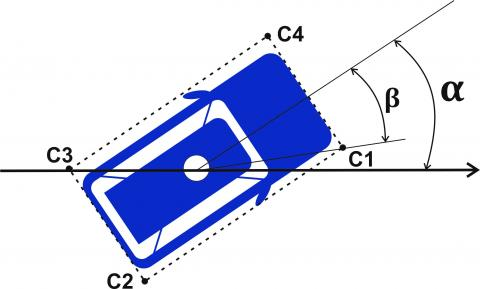Figure 8. Description of the lane change angle of autonomous vehicle

Coordinates of the 4 rectangular corners surround the vehicle VIH_AV, when changing the steering angle is as follows (Figure 8): $C_{1}\left(x_{C 1}, y_{C 1}\right), C_{2}\left(x_{C 2}, y_{C 2}\right), C_{3}\left(x_{C 3}, y_{C 3}\right) \text { and } C_{4}\left(x_{C 4}, y_{C 4}\right)$. During lane change, these $C_{1}, C_{2}, C_{3} \text { and } C_{4}$ are angular point locations that are likely to collide between the autonomous vehicle and surrounding objects.

The coordinates of the points are calculated specifically as follows:

Point C1 (xC1,yC1 )

$x_{C_{1}}=x_{\alpha v}+\frac{\sqrt{L_{\alpha v}{ }^{2}+W_{\alpha v}{ }^{2}}}{2} \cos (\alpha-\beta)$          (31)

$y_{C_{1}}=y_{a v}+\frac{\sqrt{L_{\alpha v}{ }^{2}+W_{\alpha v}{ }^{2}}}{2} \sin (\alpha-\beta)$          (32)

Point C2 (xC2,yC2 )

$x_{C 2}=x_{\alpha v}-\frac{\sqrt{L_{\alpha v}{ }^{2}+W_{a v}{ }^{2}}}{2} \cos (\alpha+\beta)$          (33)

$y_{C 2}=y_{\alpha v}-\frac{\sqrt{L_{\alpha v}{ }^{2}+W_{a v}{ }^{2}}}{2} \sin (\alpha+\beta)$          (34)

Point C3 (xC3,yC3 )

$x_{C 3}=x_{a v}-\frac{\sqrt{L_{a v}{ }^{2}+W_{a v}{ }^{2}}}{2} \cos (\alpha-\beta)$          (35)

$y_{C 3}=y_{a v}-\frac{\sqrt{L_{a v}{ }^{2}+W_{a v}{ }^{2}}}{2} \sin (\alpha-\beta)$          (36)

Point C4 (xC4,yC4)

$x_{C 4}=x_{a v}+\frac{\sqrt{L_{a v}^{2}+W_{a v}^{2}}}{2} \cos (\alpha+\beta)$          (37)

$y_{C 4}=y_{a v}+\frac{\sqrt{L_{\alpha v}^{2}+W_{\alpha v}^{2}}}{2} \sin (\alpha+\beta)$          (38)

In addition, the position of the point of collision between the autonomous vehicle and the surrounding objects is located at the edges $\left[C_{1}, C_{2}\right],\left[C_{2}, C_{3}\right],\left[C_{3}, C_{4}\right] \text { and }\left[C_{4}, C_{1}\right]$ of the surrounding rectangle, which is calculated as follows:

Edge position [C1,C2]:

$x_{\left[C_{1}, C_{2}\right]}=x_{C_{2}}+\Delta x_{[a v, r f]}$          (39)

$y_{\left[C_{1}, C_{2}\right]}=y_{B_{2}, r f}$          (40)

$\Delta x_{[a v, r f]}=\Delta y_{[a v, r f]} \cot (\alpha)q$          (41)

$\Delta y_{[a v, r f]}=y_{B_{2}, r f}-y_{C_{2}}$          (42)

Edge position [C2,C3]:

$x_{\left[C_{2}, C_{3}\right]}=x_{C_{2}}+\Delta x_{[r b, a v]}$         (43)

$y_{\left[C_{2}, C_{3}\right]}=y_{B_{4}, r b}$         (44)

$\Delta x_{[r b, a v]}=\Delta y_{[r b, a v]} \tan (\alpha)$         (45)

$\Delta y_{[r b, a v]}=y_{B_{4}, r b}-y_{C_{2}}$         (46)

Edge position [C3,C4]:

$x_{\left[C_{3}, C_{4}\right]}=x_{C_{4}}+\Delta x_{[l b, a v]}$        (47)

$y_{\left[C_{3}, C_{4}\right]}=y_{B_{3}, l b}$        (48)

$\Delta x_{[l b, a v]}=\Delta y_{[l b, a v]} \cot (\alpha)$        (49)

$\Delta y_{[l b, a v]}=y_{C_{4}}-y_{B_{3}, l b}$        (50)

Edge position [C4,C1 ]:

$x_{\left[C_{4}, C_{1}\right]}=x_{C_{1}}+\Delta x_{[a v, l f]}$          (51)

$y_{\left[C_{4}, C_{1}\right]}=y_{B_{1}, l f}$          (52)

$\Delta x_{[a v, l f]}=\Delta y_{[a v, l f]} \tan (\alpha)$          (53)

$\Delta y_{[a v, l f]}=y_{B_{1}, l f}-y_{C_{1}}$          (54)

where: Δx and Δy are the distances from the autonomous vehicle to another object in the directions OX and OY.

From (29)-(54), the real-life distance from autonomous vehicle to other objects is calculated as follows:

Distance [VIH_AV - VIH_RF]

$S_{a v, r f}=\left\{\begin{array}{ccc} x_{B_{2}, r f}-x_{C_{1}} & \text { if } & y_{C_{1}} \in\left[Y_{B_{1}, r f}, y_{B_{2}, r f}\right] \\ x_{B_{2}, r f}-x_{\left[C_{1}, C_{2}\right]} & \text { if } & y_{C_{1}}>y_{B_{2}, r f}, y_{C_{2}}<y_{B_{2}, r f} \end{array}\right.$          (55)

Distance [VIH_AV - VIH_LF]

$S_{a v, l f}=\left\{\begin{array}{ccc} x_{B_{2}, l f}-x_{C_{1}} & \text { if } & y_{C_{1}} \in\left[Y_{B_{1}, l f}, y_{B_{2}, l f}\right] \\ x_{B_{2}, l f}-x_{\left[C_{4}, C_{1}\right]} & \text { if } & y_{C_{1}}<y_{B_{1}, l f}, y_{C_{4}}>y_{B_{1}, l f} \end{array}\right.$        (56)

Distance [VIH_AV - VIH_RB]

$S_{a v, r b}=\left\{\begin{array}{ccc} x_{C_{1}}-x_{B_{4}, r b} & \text { if } & y_{C_{3}} \in\left[Y_{B_{3}, r b}, y_{B_{4}, r b}\right] \\ x_{\left[C_{2}, C_{3}\right]}-x_{B_{4}, r b} & \text { if } & y_{C_{3}}>y_{B_{4}, r b}, y_{C_{2}}<y_{B_{4}, r b} \end{array}\right.$        (57)

Distance [VIH_AV - VIH_LB]

$S_{a v, l b}=\left\{\begin{array}{ccc} x_{C_{3}}-x_{B_{3}, l b} & \text { if } & y_{C_{3}} \in\left[Y_{B_{3}, l b}, y_{B_{4}, l b}\right] \\ x_{\left[C_{3}, C_{4}\right]}-x_{B_{3}, l b} & \text { if } & y_{C_{3}}<y_{B_{3}, l b}, y_{C_{4}}>y_{B_{3}, l b} \end{array}\right.$        (58)

The safety distance model is built on the real-life distance between the rear and front vehicles, to ensure safety when performing lane change and obstacle course, the actual distance between the vehicles must be within the corresponding minimum safety distance.

In addition, due to the speed of the vehicles can change, 2 situations that will occur collision: i) the moving vehicle in front performs a slowing down/brake folding, ii) the rear vehicle moves at a greater speed than the vehicle in front.

Therefore, the safety distance model is built in combination with the real-life distance and the principle of dynamics of the vehicle. The principle of dynamics considered in this problem includes the braking time of the vehicle (including braking coordination time, braking response time and continuous braking time), acceleration and speed of the vehicle, with the following 2 models: Safety distance model when the vehicle in front performs a slowing/braking reduction and safety distance model when rear vehicle adjusts to slow down.

2.4.1 Safety distance model when the vehicle in front performs a slowing/braking reduction

With the principle of braking in each vehicle type is different [24, 25] and the maximum average braking acceleration horizontally of the direction the vehicle moves is 7m/s2 [26, 27]. As well as, the rear vehicle (VIH_BK) will perform the rear braking process of the front vehicle (VIH_FR), which has resulted in the brake speed of the rear vehicle changing will be later than the vehicle in front.

Therefore, the braking distance (Lbk ) of the rear vehicle and the braking distance (Lfr ) of the front vehicle to perform the brakes to slow down are:

$L_{b k}=v_{b k}\left(t_{r}+\frac{t_{b k}}{2}\right)-\frac{1}{24} a_{\max _{-} b k} t_{b k}^{2}+\frac{v_{b k}^{2}}{2 a_{\max _{-} b k}}$          (59)

$L_{f r}=\frac{1}{2} v_{f r} t_{f r}-\frac{1}{24} a_{\max _{-} f r} t_{f r}^{2}+\frac{v_{f r}^{2}}{2 a_{\max _{-} f r}}$          (60)

where: vbk is the speed of the vehicle behind, vfr is the speed of the vehicle front; $a_{\max _{-} b k}$ is the maximum braking acceleration of the rear vehicle, $a_{\max _{-} f r}$ is the maximum braking acceleration of the rear vehicle; $t_{r 1}, t_{b k}, t_{f r}$ are respectively the processing time periods, the time to respond to the brake operation of the rear vehicle and the time to respond to the brake operation of the vehicle in front.

Similarly, the braking distance from the autonomous vehicle to the vehicles behind the left and right is calculated as follows:

$L_{a v_{-} b k}=v_{a v}\left(t_{r}+\frac{t_{b k}}{2}\right)-\frac{1}{24} a_{\max _{-} a v} t_{b k}^{2}+\frac{v_{a v}{ }^{2}}{2 a_{\max _{-} a v}}$        (61)

$L_{R_{-} b k}=v_{R_{-} b k}\left(t_{r}+\frac{t_{b k}}{2}\right)-\frac{1}{24} a_{\max _{-} R_{-} b k} t_{b k}^{2}+\frac{v_{R_{-} b k}^{2}}{2 a_{\max _{-} R_{-} b k}}$        (62)

$L_{L_{-} b k}=v_{L_{-} b k}\left(t_{r}+\frac{t_{b k}}{2}\right)-\frac{1}{24} a_{\max _{-} L_{-} b k} t_{b k}^{2}+\frac{v_{L_{-} b k}{ }^{2}}{2 a_{\max _{-} L_{-} b k}}$        (63)

where: $a_{\max _{-} a v}, a_{\max _{-} R_{-} b k}, a_{\max _{-} L_{-} b k}$ are respectively the maximum braking acceleration of the autonomous vehicle, the vehicle behind the right and the vehicle behind the left; $v_{a v}, v_{R_{-} b k}, v_{L_{-} b k}$ are respectively the speed of the autonomous vehicle, the vehicle behind the right and the vehicle behind the left.

And the braking distances from the autonomous vehicle to the vehicles in front left and right are calculated as follows:

$L_{a v_{-} f r}=\frac{1}{2} v_{\alpha v} t_{f r}-\frac{1}{24} a_{\max _{-} a v} t_{f r}^{2}+\frac{v_{a v}^{2}}{2 a_{\max _{-} a v}}$         (64)

$L_{R_{-} f r}=\frac{1}{2} v_{R_{-} f r} t_{f r}-\frac{1}{24} a_{\max _{-} R_{-} f r} t_{f r}^{2}+\frac{v_{R_{-} f r}{ }^{2}}{2 a_{\max _{-} R_{-} f r}}$         (65)

$L_{L_{-} f r}=\frac{1}{2} v_{L_{-} f r} t_{f r}-\frac{1}{24} a_{\max _{-} L_{-} f r} t_{f r}^{2}+\frac{v_{L_{-}} f r^{2}}{2 a_{\max _{-} L_{-} f r}}$         (66)

where: $a_{\max _{-} a v}, a_{\max _{-} R_{-} f r}, a_{\max _{-} L_{-} f r}$ are respectively the maximum braking acceleration of the autonomous vehicle, the vehicle in front of the right and the vehicle in front of the left; $v_{a v}, v_{R_{-} f r}, v_{L_{-} f r}$ respectively the speed of the autonomous vehicle, the vehicle in front of the right and the vehicle in front of the left.

From (59)-(66), the safe distance model when the vehicle in front performs a speed reduction/folding brake is calculated as follows:

$L_{-} B A C K_{[b k, f r]}=L_{b k}-L_{f r}$          (67)

$L_{-} B A C K_{\left[a v, R_{fr}\right]}=L_{a v_{-} b k}-L_{R_{-} f r}$          (68)

$L_{-} B A C K_{\left[a v, L_{fr}\right]}=L_{a v_{-} b k}-L_{L_{-} f r}$          (69)

$L_{-} B A C K_{\left[R_{b k}, a v\right]}=L_{R_{-} b k}-L_{\alpha v_{-} f r}$          (70)

$L_{-} B A C K_{\left[L_{b k}, a v\right]}=L_{L_{-} b k}-L_{\alpha v_{-} f r}$          (71)

2.4.2 Safety distance model when rear vehicle adjusts to slow down

In case, if the speed of the vehicle in front vfr is greater than the speed of the vehicle behind vbk, then the actual distance between the two vehicles will increase over time and there will be no collision, which results in the minimum safety distance model that will be in value L_SPEED_[bk,fr] =0. Conversely, if the speed of the rear vehicle vbk is greater than the speed of the vehicle in front vfr, then the minimum safety distance L_SPEED_[bk,fr] =0 will be calculated as follows:

$L_{-} \operatorname{SPEED}_{[b k, f r]}=\frac{v_{b k}^{2}-v_{f r}^{2}}{2 a_{\max _{-} b k}}$          (72)

Similarly, the minimum distance values between the autonomous vehicle and the front vehicles, rear vehicles, the left and right vehicles are calculated as follows:

$\text { Case } v_{R_{-} f r} \geq v_{a v}: L_{-} \operatorname{SPEED}_{\left[\alpha v, R_{-} f r\right]}=0$          (73)

$\text { Case } v_{R_{-} f r}<v_{a v}: L_{-} S P E E D_{\left[a v, R_{-} f r\right]}=\frac{v_{a v}^{2}-v_{R_{-}} f r_{r}^{2}}{2 a_{\max _{-} a v}}$          (74)

$\text { Case } v_{L_{-} f r} \geq v_{a v}: L_{-} S P E E D_{\left[a v, L_{-} f r\right]}=0$          (75)

$\text { Case } v_{L_{-} f r}<v_{a v}: L_{-} \operatorname{SPEED}_{\left[a v, L_{-} f r\right]}=\frac{v_{a v}^{2}-v_{L_{-} f r}^{2}}{2 a_{\max _{-} a v}}$          (76)

$\text { Case } v_{R_{-} b k} \leq v_{a v}: L_{-} S P E E D_{\left[R_{-} b k, a v\right]}=0$          (77)

$\text { Case } v_{R_{-} b k}>v_{a v}: L_{-} S P E E D_{\left[R_{-} b k, a v\right]}=\frac{v_{R_{-} b k}^{2}-v_{a v}^{2}}{2 a_{\max _{-} R_{-} b k}}$          (78)

$\text { Case } v_{L_{-} b k} \leq v_{a v}: L_{-} \operatorname{SPEED}_{\left[L_{-} b k, a v\right]}=0$          (79)

$\text { Case } v_{L_{-} b k}>v_{a v}: L_{-} S P E E D_{\left[L_{-} b k, a v\right]}=\frac{v_{L_{-} b k}^{2}-v_{a v}^{2}}{2 a_{\max _{-} L_{-} b k}}$          (80)

Thus, based on the defined possible collision points, we will determine the real distance and safe distance to avoid collisions among vehicles. This safe distance model, which can ensure lane change and overtaking without collisions, or limit possible dangerous situations in the motion planning of autonomous vehicles.

2.5 Establishing a motion trajectory

The motion trajectory in this paper is established with phases taken as follows:

Phase 1: Determining the position of the objects (position of an autonomous vehicle and surrounding vehicles)

Processing GPS positioning data and sensor systems, locating objects on the Cartesian coordinate system, calculating the distance among objects.

Phase 2: Building an initial trajectory

Based on the information obtained from phase 1, we build the initial motion trajectory using a quintic polynomial curve to perform lane change or obstacle course.

Phase 3: Identifying the target function and checking the constrain condition

We consider the constraint conditions of safe distance and identify as a target function to establish an optimal nonlinear model to make decisions on how to change lanes or overtake obstacles to ensure safety, avoid collisions and ensure smooth and slippery trajectory.

Phase 4: Lane change trajectory planning or obstacle overtaking

The vehicle determines the parameters to create the final motion trajectory. If it finds a possible solution, it will perform lane change planning or obstacle overtaking. In the opposite case, if there is not a possible solution, the autonomous vehicle will continue to move on the current lane and continue to repeat the steps until it finds a possible trajectory.

3. Experimental Results

To test and evaluate the proposed solution, we conduct an experimental simulation of processes in a Matlab environment. At the same time, in order to ensure objectivity and reliability when evaluating, we conduct simulations with different scenarios, including obstacle avoidance scenarios and free lane change scenarios, the speed of movement of vehicles in the simulation is conducted with 2 values including 40 km/h  and 60 km/h. The experimental road is a straight road, with width according to lane standards, $D_{\text {lane_width }}=3.5 \mathrm{~m}$ with this standard size, the maximum speed of movement is 80 km/h and the two autonomous cannot overtake each other if the latter does not switch to the next lane; The distance from the autonomous vehicle to the vehicle in front when starting to perform lane change is 40 m.

The input variables will be processed using experimental data obtained from GPS devices, the process of selecting data conducted from the driving behavior of drivers that meet the conditions set by the problem. Therefore, the input data ensures the creation of a safe motion process, the reference path and the left $\mathrm{b}_{1}$ and right br boundary lines are designed and determined in accordance with the road system. During the experiment, the calculations use the SI measurement system, the trajectory transfer to the I/O controller with a room tissue time cycle of 0.005 s.

The specific parameters of the objects (including an autonomous vehicle and other vehicles in traffic) are used in the simulation as follows: Wheelbase of the vehicle L=4600mm, wheel width of the vehicle W=1700mm, minimum rotation radius r=5300mm (the measurement calculated based on the way the vehicle rotates in place, then calculated from the center of the circle to the outermost wheel), the speed of the vehicle $v \in[0,80] \mathrm{km} / \mathrm{h}$, acceleration of the vehicle $a \in[-3,3] \mathrm{m} / \mathrm{s}^{2}$, jerk of the vehicle $j \in[-3,3] \mathrm{m} / \mathrm{s}^{3}$ , number of time intervals $I=30$.

Scenario 1: At the beginning, the autonomous vehicle is moving in lane 1, located behind the vehicle in the next lane (lane 2). The autonomous vehicle will perform lane change to overtake the vehicle in front (Figure 9). To change lane successfully, it must pass the vehicle in the next lane and changes lane, then change the next lane to overtake the obstacle vehicle in front. The original coordinates of the vehicles in the Cartesian coordinate system (x,y) are as follows: $V I H_{-} A V(85,2), \quad V I H_{-} R B(20,1.5), \quad V I H_{-} L B(130,5), V I H_{-} R F(180,2) \text { and } V I H_{-} L F(250,5)$.

## 9.jpg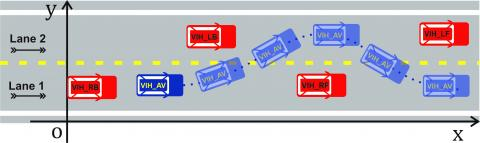Figure 9. Simulation scenario 1

Scenario 2: Similar to Scenario 1, the autonomous vehicle is moving in lane 1 and is located in front of the vehicle in the next lane (lane 2). It will perform a lane change to overtake the vehicle in front (Figure 10). The original coordinates of the vehicles in the Cartesian coordinate system (x,y) are as follows: $V I H_{-} A V(120,2), \quad I H_{-} R B(20,1.5) , \quad V I H_{-} L B(60,5), \quad V I H_{-} R F(180,2) \text { and } V I H_{-} L F(250,5)$.

To verify the feasibility and effectiveness of the solution, the simulation process is conducted with 100 cases of lane change and obstacle overtaking. The initial trajectories are randomly initiated.

## 10.jpgFigure 10. Scenario 2 simulation

Through the proposed model, the experimental results are as follows:

Scenario 1: 80 out of 100 test cases were determined to find a viable obstacle trajectory with lane change and obstacle overtaking times in the range of 7-10 s (Figure 9). In which: the shortest time period is $t_{\min }=3.2 \mathrm{~s}$, the longest period is $t_{\min }=13.4 \mathrm{~s}$ and the average time period for the cases is $t_{\text {mean }}=7.8 \mathrm{~s}$, the change speed $\Delta v=\left|v_{\max }-v_{0}\right|=4.16 \mathrm{~km} / \mathrm{h}$, maximum acceleration $\left|a_{\max }\right|=2.78 / \mathrm{s}^{2}$, maximum jerk $\left|j_{\max }\right|=2.95 / s^{3}$.

Scenario 2: It was identified that 85 out of 100 test cases found a viable obstacle trajectory with lane change and obstacle overtaking times in the range of 5-9s (Figure 10). In which: the shortest period of time is $t_{\min }=3.5 \mathrm{~s}$, the longest period is $t_{\min }=12.5 \mathrm{~s}$  and the average time period for the case is $t_{\text {mean }}=6.2 \mathrm{~s}$ , the change speed $\Delta v=\left|v_{\max }-v_{0}\right|=3.21 \mathrm{~km} / \mathrm{h}$ , maximum acceleration $\left|a_{\max }\right|=1.42 / \mathrm{s}^{2}$ , maximum jerk $\left|j_{\max }\right|=1.81 \mathrm{~m} / \mathrm{s}^{3}$.

With the input parameters of the vehicle model and the experimental results in 2 scenarios, we can see that the average time to change lanes and overtake obstacles in scenario 1 $\left(t_{\text {mean }}=7.8 \mathrm{~s}\right)$  is greater than the corresponding value in scenario 2 $\left(t_{\min }=3.5 s\right)$  since autonomous vehicle must have time to overtake the vehicle in the next lane. At the same time, with the values of speed change, maximum acceleration and maximum jerk are located at a low level have ensured the lane change trajectory and obstacle overtaking to achieve smooth and slippery criteria.

If the traffic participation status of the surrounding vehicles changes (increased/decreased velocity, lane change), the trajectory of motion of the autonomous vehicle will be reset to avoid a possible collision. The reset of motion will be considered as the original trajectory, the process of changing lanes to overtake and avoid obstacles can still be created using the model proposed in this study.

Thus, with the experimental process as presented has demonstrated the feasibility of the solution, it is possible to respond in most traffic situations, including emergency situations with the process of changing the speed or changing lanes of other vehicles involved in the surrounding traffic.

4. Conclusion

This article proposes a solution for lane change planning and obstacle overtaking for autonomous vehicles by creating an trajectory in the Cartesian coordinate system (x,y) connecting from the original position to the target position of the traffic-taking state. In which the optimal nonlinear model built including the operating status of autonomous vehicles and other road participants has created different lane-changing and obstacle-taking trajectories. At the same time, the trajectory created during lane change must ensure the element of collision avoidance, smoothness, slipperiness, which are the criteria to create a constraint set for the optimal trajectory selection process.

Results of experimental simulations in Matlab environments have demonstrated that autonomous vehicles can effectively avoid potential collisions with the process of selecting safe trajectories and conditions by building a minimum safe distance model to avoid collisions. Finally, lane change trajectory and obstacle overtaking can be established in different traffic situations.

The main idea of this technical solution will support the design of a safe stop-alone autonomous vehicle, no matter what the current control of the vehicle is. The experimental simulation section with the scenarios given shows that safety factors can be achieved by calculation to consider all possible possibilities of other vehicles participating in traffic.

In the future, to increase the reliability of this solution, the simulated settings will be transferred to the real-life environment with the experimental vehicle fully equipped with sensors. And when it is experimented in real life, it will add some factors to analyze the stability of the system so that the traffic behavior of the vehicles will be predicted more accurately. The widespread implementation of this solution for semi-autonomous or fully autonomous vehicles in vehicle control systems will be able to minimize the large number of damages as well as create a safe motion plan for the future.

References

 Childress, S., Nichols, B., Charlton, B., Coe, S. (2015). Using an activity-based model to explore the potential impacts of automated vehicles. Transportation Research Record, 2493(1): 99-106. https://doi.org/10.3141/2493-11

 Yang, L., Song, D., Xiao, J., Han, J., Yang, L., Cao, Y. (2015). Generation of dynamically feasible and collision free trajectory by applying six-order Bezier curve and local optimal reshaping. In 2015 IEEE/RSJ International Conference on Intelligent Robots and Systems (IROS), Hamburg, Germany, pp. 643-648. https://doi.org/10.1109/IROS.2015.7353440

 Simba, K.R., Uchiyama, N., Sano, S. (2016). Real-time smooth trajectory generation for nonholonomic mobile robots using Bézier curves. Robotics and Computer-Integrated Manufacturing, 41: 31-42. https://doi.org/10.1016/j.rcim.2016.02.002

 Choe, R., Puig-Navarro, J., Cichella, V., Xargay, E., Hovakimyan, N. (2016). Cooperative trajectory generation using Pythagorean hodograph Bézier curves. Journal of Guidance, Control, and Dynamics, 39(8): 1744-1763. https://doi.org/10.2514/1.G001531

 Zong, X., Sun, Q., Yao, D., Du, W., Tang, Y. (2019). Trajectory planning in 3D dynamic environment with non-cooperative agents via fast marching and Bézier curve. Cyber-Physical Systems, 5(2): 119-143. https://doi.org/10.1080/23335777.2019.1590460

 Rousseau, G., Maniu, C.S., Tebbani, S., Babel, M., Martin, N. (2019). Minimum-time B-spline trajectories with corridor constraints. Application to cinematographic quadrotor flight plans. Control Engineering Practice, 89: 190-203. https://doi.org/10.1016/J.CONENGPRAC.2019.05.022

 Liu, H., Lai, X., Wu, W. (2013). Time-optimal and jerk-continuous trajectory planning for robot manipulators with kinematic constraints. Robotics and Computer-Integrated Manufacturing, 29(2): 309-317. https://doi.org/10.1016/j.rcim.2012.08.002

 Ziegler, J., Stiller, C. (2009). Spatiotemporal state lattices for fast trajectory planning in dynamic on-road driving scenarios. In 2009 IEEE/RSJ International Conference on Intelligent Robots and Systems, MO, USA, pp. 1879-1884. https://doi.org/10.1109/IROS.2009.5354448

 Huang, J., Hu, P., Wu, K., Zeng, M. (2018). Optimal time-jerk trajectory planning for industrial robots. Mechanism and Machine Theory, 121: 530-544. https://doi.org/10.1016/j.mechmachtheory.2017.11.006

 Rodríguez, L., Balampanis, F., Cobano, J.A., Maza, I., Ollero, A. (2017). Energy-efficient trajectory generation with spline curves considering environmental and dynamic constraints for small UAS. In 2017 IEEE/RSJ International Conference on Intelligent Robots and Systems (IROS), Vancouver, Canada, pp. 1739-1745. https://doi.org/10.1109/IROS.2017.8205987

 Yang, J., Hu, Q., Ding, H. (2016). A two-stage CNC interpolation algorithm for corner smoothing trajectories with geometric error and dynamics constraints. Procedia CIRP, 56: 306-310. https://doi.org/10.1016/j.procir.2016.10.022

 Liu, C., Sun, B., Li, F. (2017). Acceleration planning based anti-swing and position control for double-pendulum cranes. In 2017 29th Chinese Control and Decision Conference (CCDC), Chongqing, China, pp. 5671-5675. https://doi.org/10.1109/CCDC.2017.7978176

 Chen, W.C., Chen, C.S., Lee, F.C., Chen, L.Y. (2019). High speed blending motion trajectory planning using a predefined absolute accuracy. The International Journal of Advanced Manufacturing Technology, 104(5): 2179-2193. https://doi.org/10.1007/s00170-019-03973-y

 Yin, W., Sun, L., Wang, M., Dong, S., Liu, J. (2016). Residual vibration suppression for series elastic actuator based on phase plane analysis and trajectory tracking. In 2016 35th Chinese Control Conference (CCC), Chengdu, China, pp. 4453-4458. https://doi.org/10.1109/ChiCC.2016.7554045

 Xia, Z., Zhang, Z., Zhou, C., Hong, L. (2016). A method for industrial robot manipulators trajectory planning based on piecewise continuous function. In Proceedings of the International Conference on Artificial Intelligence and Robotics and the International Conference on Automation, Control and Robotics Engineering, Kitakyushu, Japan, pp. 1-5. https://doi.org/10.1145/2952744.2952760

 Wei, C., Wang, Y., Asakura, Y., Ma, L. (2021). A nonlinear programing model for collision-free lane-change trajectory planning based on vehicle-to-vehicle communication. Journal of Transportation Safety & Security, 13(9): 936-956. https://doi.org/10.1080/19439962.2019.1701165

 Yang, D., Zheng, S., Wen, C., Jin, P.J., Ran, B. (2018). A dynamic lane-changing trajectory planning model for automated vehicles. Transportation Research Part C: Emerging Technologies, 95: 228-247. https://doi.org/10.1016/j.trc.2018.06.007

 Bai, H., Shen, J., Wei, L., Feng, Z. (2017). Accelerated lane-changing trajectory planning of automated vehicles with vehicle-to-vehicle collaboration. Journal of Advanced Transportation, 2017: 8132769. https://doi.org/10.1155/2017/8132769

 Nilsson, J., Brännström, M., Coelingh, E., Fredriksson, J. (2016). Lane change maneuvers for automated vehicles. IEEE Transactions on Intelligent Transportation Systems, 18(5): 1087-1096. https://doi.org/10.1109/TITS.2016.2597966

 Zhang, S., Deng, W., Zhao, Q., Sun, H., Litkouhi, B. (2013). Dynamic trajectory planning for vehicle autonomous driving. In 2013 IEEE International Conference on Systems, Man, and Cybernetics, Manchester, UK, pp. 4161-4166. https://doi.org/10.1109/SMC.2013.709

 Su, T., Cheng, L., Wang, Y., Liang, X., Zheng, J., Zhang, H. (2018). Time-optimal trajectory planning for delta robot based on quintic Pythagorean-hodograph curves. IEEE Access, 6: 28530-28539. https://doi.org/10.1109/ACCESS.2018.2831663

 Tho, Q.H., Phap, H.C., Phuong, P.A. (2020). A solution applying the law on road traffic into a set of constraints to establish a motion trajectory for autonomous vehicle. Advances in Science, Technology and Engineering Systems Journal, 5(3): 450-456. https://doi.org/10.25046/aj050356

 United Nations (2020). Law of the Sea Bulletin, No. 97, ISBN-10: 9211338743.

 Xiong, H., Zhu, X., Zhang, R. (2018). Energy recovery strategy numerical simulation for dual axle drive pure electric vehicle based on motor loss model and big data calculation. Complexity, 2018: 4071743. https://doi.org/10.1155/2018/4071743

 Rehkopf, J. (2008). Automobile Brake Systems, Pearson Prentice Hall, Upper Saddle River, NJ, USA.

 Lian, J.Y., Hua, X.Y. (2005). Study of mathematics model for rear end collision avoidance system. China Journal of Highway and Transport, 18(3): 123-126.

 Jiang, N.H., Yu, J.G. (2010). Study on rear-end avoidance model of highway based on RBF neural network. Forest Engineering, 26(5): 60-65. https://doi.org/10.16270/j.cnki.slgc.2010.05.023